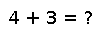# isequal

isequal(x, y)

Similar to `==`, except treats all floating-point `NaN` values as equal to each other, and treats `-0.0` as unequal to `0.0`. The default implementation of `isequal` calls `==`, so if you have a type that doesn't have these floating-point subtleties then you probably only need to define `==`.

`isequal` is the comparison function used by hash tables (`Dict`). `isequal(x,y)` must imply that `hash(x) == hash(y)`.

This typically means that if you define your own `==` function then you must define a corresponding `hash` (and vice versa). Collections typically implement `isequal` by calling `isequal` recursively on all contents.

Scalar types generally do not need to implement `isequal` separate from `==`, unless they represent floating-point numbers amenable to a more efficient implementation than that provided as a generic fallback (based on `isnan`, `signbit`, and `==`).

## Examples

Checking you are not a robot: